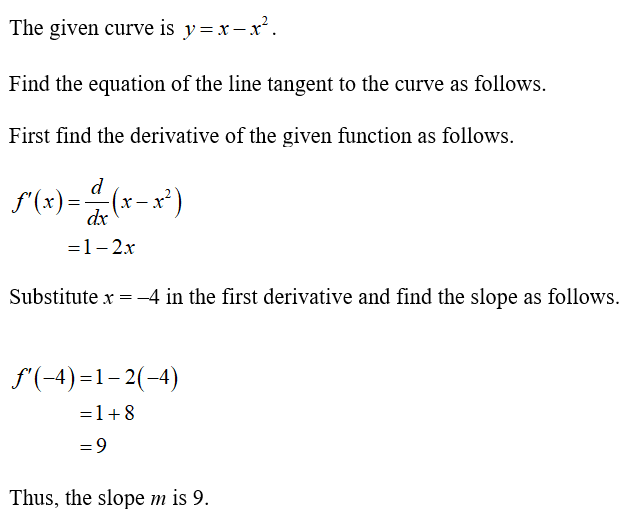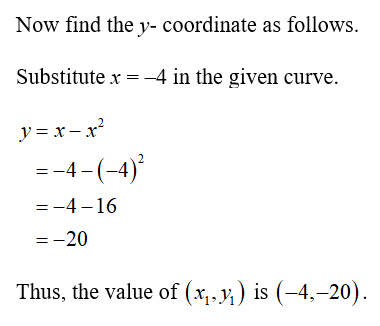# Find the equation of the line tangent to the curve y=x-x^2 (x squared) at x=-4

Question
1 views

Find the equation of the line tangent to the curve y=x-x^2 (x squared) at x=-4

check_circle

Step 1Step 2...

### Want to see the full answer?

See Solution

#### Want to see this answer and more?

Solutions are written by subject experts who are available 24/7. Questions are typically answered within 1 hour.*

See Solution
*Response times may vary by subject and question.
Tagged in